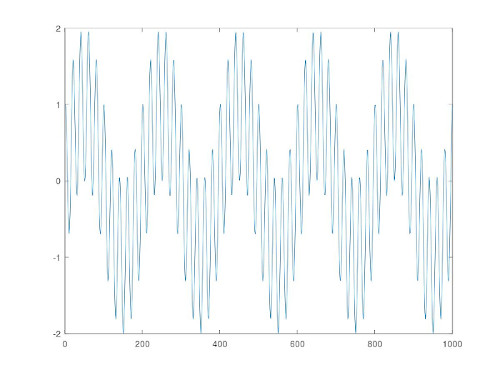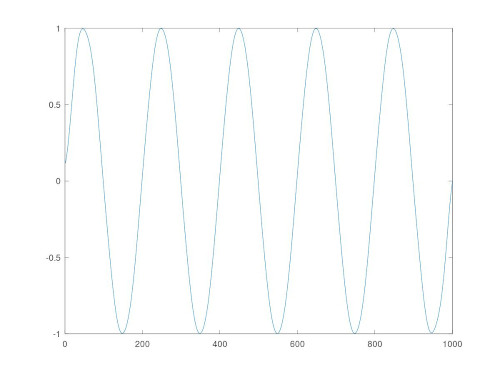## November – Digital Filtering

Let's construct a simple digital band-pass filter based in the sinc filter.

As everyone know, the sinc function is the impulse response of a low-pass filter. That is, the convolution of a signaland the sinc functionwill produce a low-pass filtered signalwithout frequencies higher than. Similarly, the functionwhererepresents the Dirac delta function, is the frequency response of the ideal high-pass filter of frequency.

Heuristically, we can right away derive the discrete versions of the impulse responses:for the low-pass filter, andfor the high-pass filter. The variableis the sampling frequency andis the Kronecker delta.

Now, having a discrete signal at hand, band-pass filtering is (in the simplest approach) just a matter of discrete convolution of the signal with the functions above. The following octave code does the job.

##Sinc band-pass filter. f0 = B_L/fs, f1 = B_H/fs

function filtered = sincfilter(signal, f0, f1)
if(mod(length(signal), 2) != 0) #Check if the signal length is even or odd.
signal = signal(1 : length(signal) - 1);
end

M = 10000; #Increase this to increase accuracy.
sincF = zeros(1, M);
for m =  -M/2 + 1 : 1 : M/2
if(!(m  == 0))
sincF(m + M/2) = sin(2*pi*f1*m)./(pi*m);
else
sincF(m + M/2) = 2*f1;
end
end

sincH = zeros(1,M);
for m =  -M/2 + 1 : 1 : M/2
if(!(m  == 0))
sincH(m + M/2) = -sin(2*pi*f0*m)./(pi*m);
else
sincH(m + M/2) = -2*f0 + 1;
end
end

##Plot stuff.
figure(1)
plot(sincF)
figure(2)
plot(signal)

tic
filtered = conv(signal, sincF, "same");
filtered = conv(filtered, sincH, "same");
toc

figure(3)
plot(filtered)
endFor more accuracy and effectiveness, one should use windowed or recursive filters. You can check in the references for more sophisticated methods!

References:

• Steven W. Smith, “Digital Signal Processing – A Practical Guide for Engineers and Scientists”, Elsevier Science, 2003.